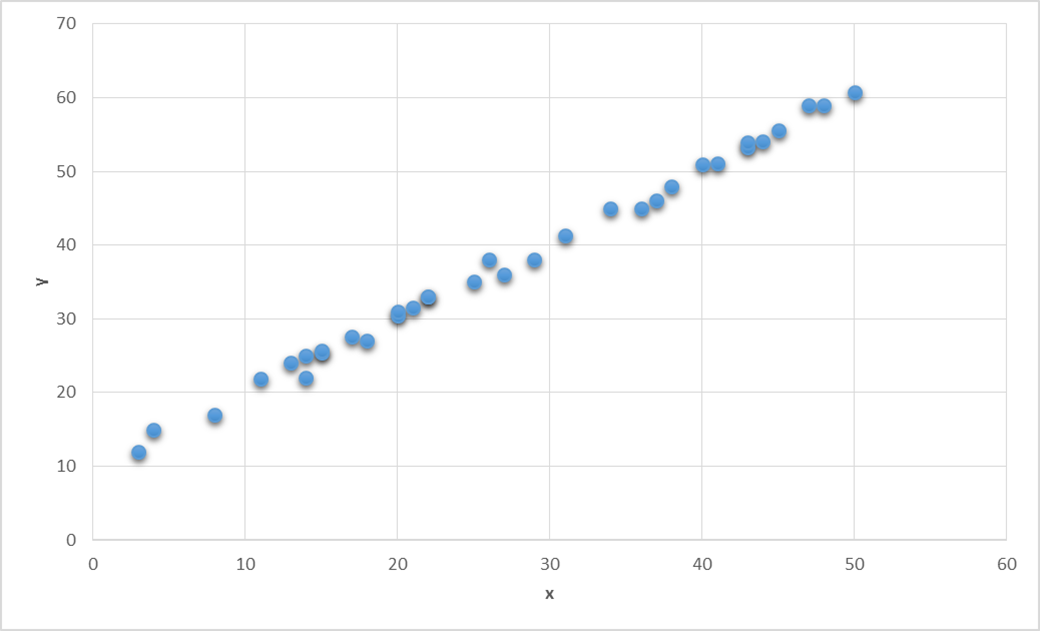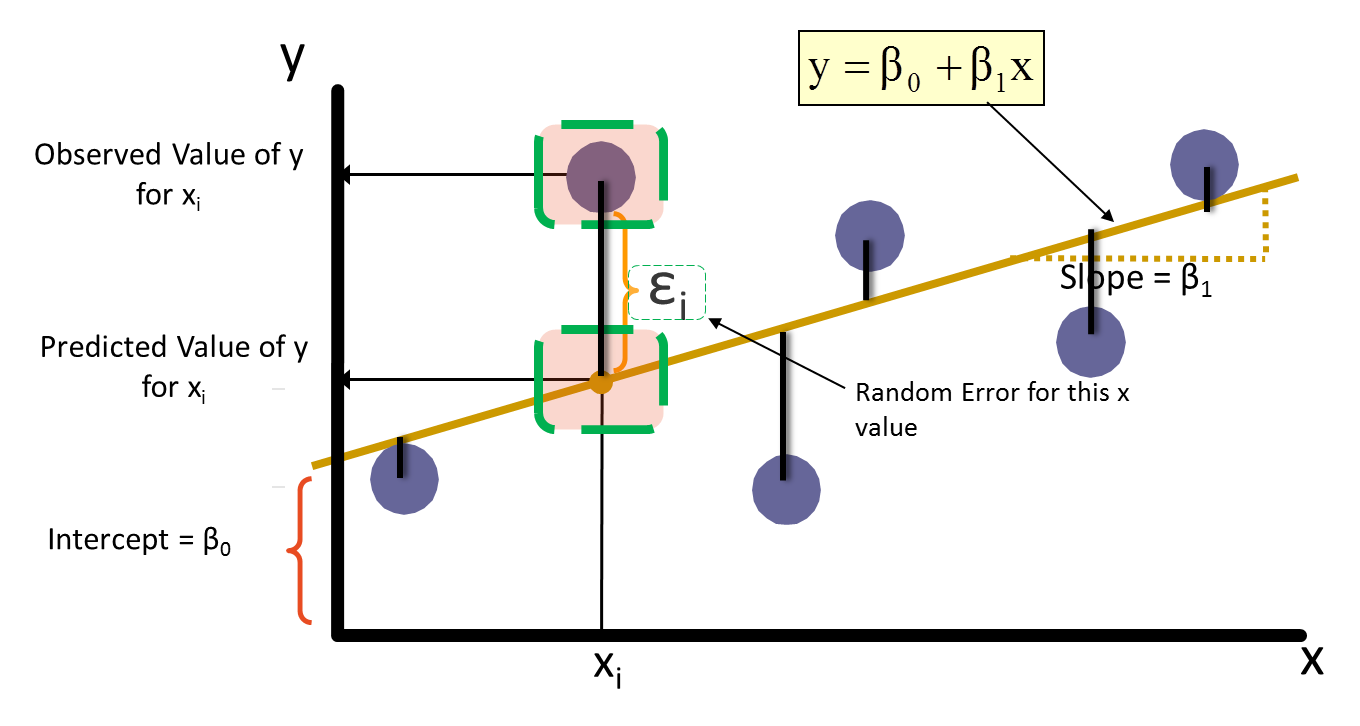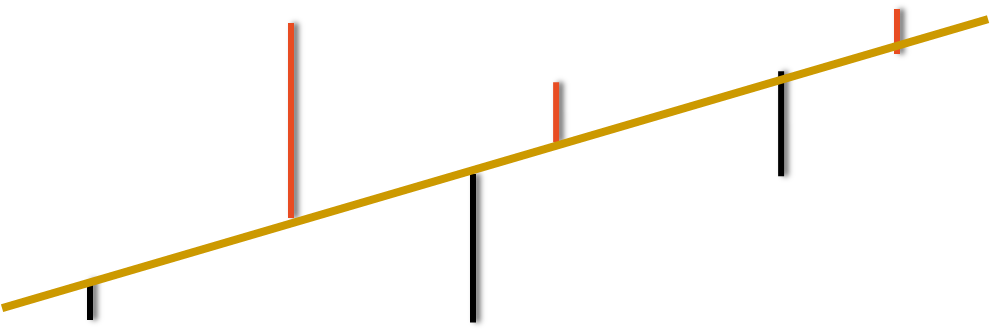• No products in the cart.

# 204.1.2 Regression in Python

##### From Correlation to Regression

Link to the previous post : https://statinfer.com/204-1-1-correlation-in-python/

In the last post we went through the concept of Correlation and implemented it using python on a dataset.

In this post we will walk from correlation to Regression.

### From Correlation to Regression

• Correlation is just a measure of association
• It can’t be used for prediction.
• Given the predictor variable, we can’t estimate the dependent variable.
• In the air passengers example, given the promotion budget, we can’t get an estimated value of passengers
• We need a model, an equation, a fit for the data.
• That is known as regression line

### What is Regression

• A regression line is a mathematical formula that quantifies the general relation between a predictor/independent (or known variable x) and the target/dependent (or the unknown variable y)
• Below is the regression line. If we have the data of x and y then we can build a model to generalize their relation
y=β0+β1x
- What is the best fit for our data?
- The one which goes through the core of the data
- The one which minimizes the error

### Regression### Regression Line fitting### Error### Minimizing the error

• The best line will have the minimum error.
• Some errors are positive and some errors are negative. Taking their sum is not a good idea.
• We can either minimize the squared sum of errors Or we can minimize the absolute sum of errors.
• Squared sum of errors is mathematically convenient to minimize.
• The method of minimizing squared sum of errors is called least squared method of regression.

### Least Squares Estimation

• X: x1, x2, x3,… xn
• Y: y1, y2, y3,… \$y_n
• Imagine a line through all the points
• Deviation from each point (residual or error)
• Square of the deviation
• Minimizing sum of squares of deviation
e2=(yy^)2
e2=(y(β0+β1x))2
• β0 and β1 are obtained by minimizing the sum of the squared residuals

The next post is a practice session on regression line fitting.

Link to the next post : https://statinfer.com/204-1-3-practice-regression-line-fitting/

21st June 2017